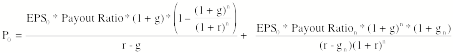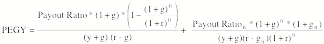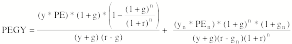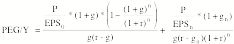Solution to Weekly Challenge 7

Using the equation for the two-stage dividend discount model, isolate the determinants of the PEGY ratio. The PEGY ratio is

PEGY = PE/ (Expected growth rate + Dividend Yield)

Dividend yield = DPS0/ P0

The equation for the two-stage dividend discount model is reproduced below:Using the equation that you end up with for the PEGY ratio estimate the PEGY ratio for a hypothetical firm (make up a growth rate, payout ratio and beta) and construct a perfect under valued firm.

Let the dividend yield=y. If you divide through the equation above by EPS0 first and then by (g + y), you get an equation that is almost intractable (in terms of simplification):I tried different formulations and came to the conclusion that the PEGY ratio was a function of the dividend payout ratio, the growth rate, the cost of equity and the level of the dividend yield. Other things remaining equal, higher dividend yield companies will have lower PEGY ratios than higher dividend yield companies. You could write the dividend payout ratio in terms of the dividend yield:

Dividend payout ratio = DPS/EPS = (DPS/P) (P/EPS) = Yield * PE

Substituting back into the equation, you getAt this stage, I gave up and cheated and tried PE/(g*y), a variation of the PEGY ratio and got the following:What does it all mean? The PEG/Y ratio for firm will be a function of both its current PE and forward PE ratios. All in all, you have a recipe for a complete and total mess…

P.S: To get this solution write out the payout ratio as DPSn/EPSn and divide both sides of the equation by the dividend yield (DPS0/P0